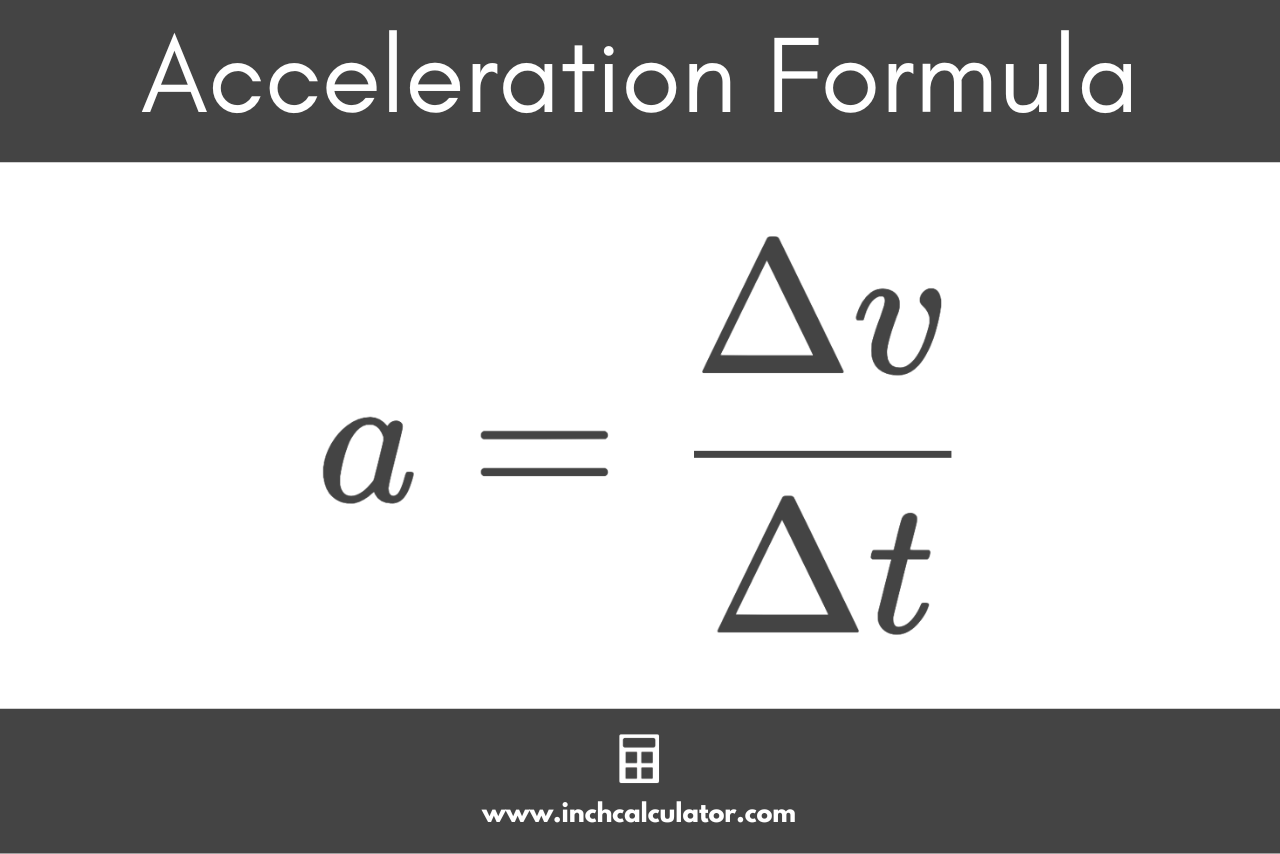# Acceleration Calculator

Use our acceleration calculator to find acceleration using velocity and time.

Calculate:

## Acceleration:

-

### Acceleration Formula

a=\frac{v_{t}-v_{0}}{t}
Learn how we calculated this below

## How to Calculate Acceleration

Acceleration is a fundamental concept in physics and represents the rate of change of velocity with respect to time. Whether it’s a car speeding up, a ball being thrown, or a plane taking off, the principle of acceleration is at work.

Essentially, acceleration tells us how much the velocity of an object changes over a specific period. You can calculate acceleration if you know the initial and final velocity of an object and the time it takes to reach the final velocity.

### Acceleration Formula

The acceleration formula is:

a=\frac{v_{t}-v_{0}}{t}

Thus, the acceleration a of an object is equal to the difference of the final velocity vt and the initial velocity v0, divided by the time duration t.

The difference of the final velocity and initial velocity is sometimes expressed as the change in velocity Δv and the time duration is sometimes expressed as Δt.

So, the formula to calculate acceleration can also be expressed as:

a=\frac{\Delta v}{\Delta t}

The acceleration a of an object is equal to the change in velocity Δv divided by the change in time Δt.For example, let’s calculate the acceleration of a car that accelerates from an initial velocity of 20 m/s to a final velocity of 50 m/s over 5 seconds.

a=\frac{20 m/s-50 m/s}{5 s}
a=\frac{30 m/s}{5 s}
a=6m/s^{2}

## Types of Acceleration

There are a few different types of acceleration:

• Uniform Acceleration: This is when an object’s velocity changes by the same amount over equal intervals of time. A car going from 0 to 60 mph in 10 seconds and then from 60 to 120 mph in the next 10 seconds is experiencing uniform acceleration.
• Non-uniform Acceleration: In this type, the rate of change of velocity is not constant over time. If that same car went from 0 to 50 mph in 10 seconds and then from 50 to 90 mph in the next 10 seconds, it would be experiencing non-uniform acceleration.
• Tangential or Linear Acceleration: This refers to the acceleration of an object moving in a straight line.
• Radial or Centripetal Acceleration: This is experienced by an object moving in a circular path. It acts towards the center of the circle.

## Common Acceleration Measurement Units

Acceleration is typically measured in meters per second squared (m/s²), feet per second squared (ft/s²), or g-force (g).

In the realm of automotive performance, you might also hear about “zero to sixty” times as a way to describe how quickly a vehicle can accelerate, but it’s not a formal unit of acceleration.

### What is G-Force

G-force, or gravitational force equivalent, is the force of gravity on an object. Earth’s gravitational acceleration is approximately 9.81 m/s².

This is often used as a baseline for comparing other accelerations. For instance, astronauts during a space shuttle launch might experience accelerations up to 3g, meaning three times the force of Earth’s gravity.